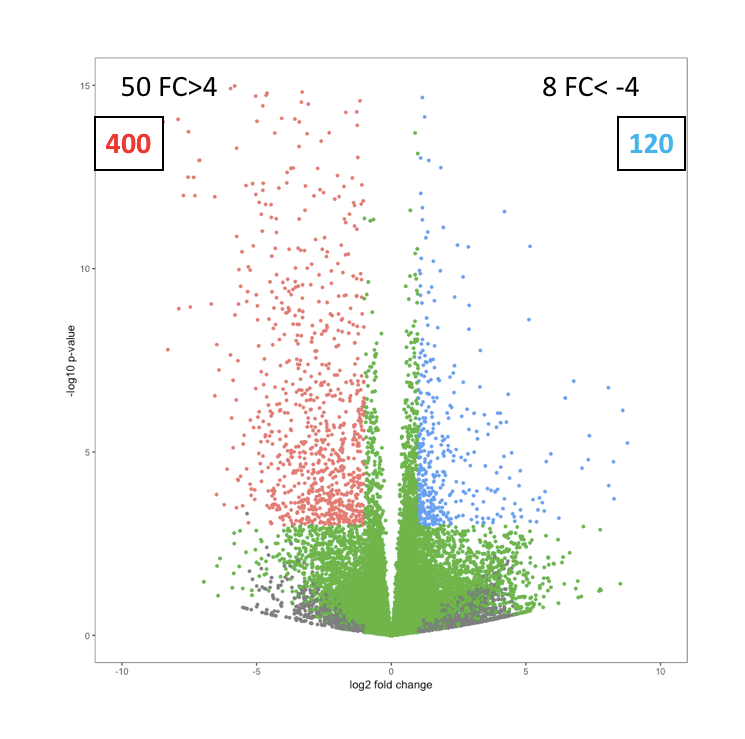Biostar Beta. Not for public use.
How to add stats. to volcano plot?
2
Entering edit mode
20 months ago
Royan Institute, Tehran, Iran

Hi

How can I add statistics to volcano plot in R for gene expression? Statistics like up and down number of genes and highly up and down genes.

use example code for that :

``````library(ggplot2)
fold_changes <- c(rnorm(20000, 0, 2))
pvalues <- runif(n=20000, min=1e-50, max=.1)
dif <- data.frame(fc =fold_changes,pv =pvalues)
dif\$thershold <- ifelse(dif\$fc > 1 & dif\$pv < 0.01, "red",
ifelse(dif\$fc < -1 & dif\$pv < 0.01, -1, "blue"))
ggplot(data=dif, aes(x=fc, y=-log10(pv))) +
geom_point( size=1 ,aes(color=as.factor(thershold))) +
theme(legend.position = "none") +
xlim(c(-10, 10)) + ylim(c(0, 15)) +
xlab("log2 fold change") + ylab("-log10 p-value")  + theme_bw()+
theme(panel.grid.major = element_blank(),
panel.grid.minor = element_blank(),)
``````

Like below image:4
Entering edit mode
13 months ago
India

try annotate:

``````ggplot(data=dif, aes(x=fc, y=-log10(pv))) +
geom_point( size=1 ,aes(color=as.factor(thershold))) +
xlim(c(-10, 10)) + ylim(c(0, 6)) +
xlab("log2 fold change") +
ylab("-log10 p-value")  +
annotate("label", x =c(-8,5), y = 4.75, label = c("400","120"), col=c("red","steelblue"))+
annotate("text", x =c(-8,5), y = 5, label = c("50 FC>4","8FC <-4"))+
theme_bw()+
theme(panel.grid.major = element_blank(),
panel.grid.minor = element_blank(),
legend.position = "none")
``````By editing x and y coordinates, you can move the text around graph, in x and y directions.

0
Entering edit mode

Going one step further, we could calculate the x-y positions for label and text, instead of hard coding them.

0
Entering edit mode

Hi

How can we choose proper x-y coordination in code like my example image?

Thanks

0
Entering edit mode

thank you so much. It is helpful.

1
Entering edit mode

No problem. You have posted input data, code and output expected. This helps in understanding the issue better and resolve it faster. ahmad.moousavi